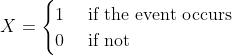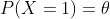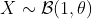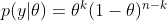Definition

In computer science, most algorithms run to completion: they provide a single answer after performing some fixed amount of computation. But nowadays, in machine learning generation, the models take a long time to train and predict the result, the user may wish to terminate the algorithm before completion. That’s where anytime algorithm came in, an algorithm that can return a valid solution even if it is interrupted before it ends. The longer it keeps running, the better solution the user gets.

What makes anytime algorithms unique is their ability to return many possible outcomes for any given input. An anytime algorithm uses many well-defined quality measures to monitor progress in problem-solving and distributed computing resources. While this may sound like dynamic programming, the difference is that it is fine-tuned through random adjustments, rather than sequential.

Algorithm prerequisites

Initialize: While some algorithms start with immediate guesses, anytime algorithm take more calculated approach and have a start–up period before making any guesses

Growth direction: How the quality of the program’s “output” or result, varies as run time

Growth rate: Amount of increase with each step. Does it change constantly or unpredictably?

End condition: The amount of runtime needed

Case studies

• Clustering with time series

In clustering, we must compute distance or similarity between pairs of time series and we also have a lot of measurements. As many types of research proved that dynamic time warping (DTW) is more robust than others, but the complexity O(N2) of DTW makes computation more trouble.

With anytime algorithm, the life is easier than ever. Below is pseudocode for anytime clustering algorithm.

Algorithm [Clusters] = AnytimeClustering(Dataset)
3.  Disp("Setup is done, interruption is possible")
4.  O = OrderToUpdateDTW(Dataset)
5.  For i = 1:Length(O)
7.     if UserInterruptIsTrue()
9.        if UserTerminateIsTrue()
10.          return
11.       endif
12.    endif
13. endfor

Firstly, we need to approximate the distance matrix of the dataset.

Secondly, we do cluster to get the very basic result.

Thirdly, we make some heuristic to find the optimal solution to update the distance matrix, after each updating, we get a better result than before.

Lastly, if the algorithm keeps running longer, it will finish the task and we can get the final optimal output.

• Learning optimal Bayesian networks

Another common example for anytime algorithm is searching optimal output in Bayesian networks. As we know that Bayesian network is NP–hard. If we have too many variables or events, the algorithm will fail due to limited time and memory. That’s why the anytime weighted A* algorithm is often applied to find an optimal result.

Weighted A* algorithm use this cost function to minimize

f(n) = g(n) + ε * h(n)

where n is the last node on the graph, g(n) is the cost of the path from the start node to n, and h(n) is a heuristic that estimates the cost of the cheapest path from n to the last node, ε gradually lowers to 1. The anytime algorithm bases on this cost function to stop and continue at any time.

Bayesian estimator of the Bernoulli parameter

In this post, I will explain how to calculate a Bayesian estimator. The taken example is very simple: estimate the parameter θ of a Bernoulli distribution.

A random variable X which has the Bernoulli distribution is defined aswithIn this case, we can write.

In reality, the simplest way to estimate θ is to sample X, count how many times the event occurs, then estimate the probability of occurring events. This is exactly what the frequentists do.

In this post, I will show how do the Bayesian statisticians estimate θ. Although this doesn’t have a meaningful application, it helps to understand how do Bayesian statistics work. Let’s start.

The posterior distribution of θ

Denote Y as the observation of the event. Given the parameter θ, if we sample the event n time, then the probability that the event occurs k time is (this is called the probability density function of Bernoulli )In Bayesian statistics, we would like to calculate

By using the Bayesian formula, we haveWith the prior distribution of theta as a Uniform distribution, p(θ) = 1, and it is easy to prove thatwhere Γ is the Gamma distribution. Hence, the posterior distribution isFortunately, this is the density function of the Beta distribution:We use the following properties for evaluating the posterior mean and variance of theta.

If, thenSimulation

In summary, the Bayesian estimator of theta is the Beta distribution with the  mean and variance as above. Here are the Python codes for simulating data and estimating theta

def bayes_estimator_bernoulli(data, a_prior=1, b_prior=1, alpha=0.05):
'''Input:
data is a numpy array with binary value, which has the distribution B(1,theta)    a_prior, b_prior: parameters of prior distribution Beta(a_prior, b_prior)    alpha: significant level of the posterior confidence interval for parameter    Model:
for estimating the parameter theta of a Bernoulli distribution    the prior distribution for theta is Beta(1,1)=Uniform[0,1]    Output:
a,b: two parameters of the posterior distribution Beta(a,b)
pos_mean: posterior estimation for the mean of theta
pos_var: posterior estimation for the var of theta'''
n = len(data)
k = sum(data)
a = k+1
b = n-k+1
pos_mean = 1.*a/(a+b)
pos_var = 1.*(a*b)/((a+b+1)*(a+b)**2)
## Posterior Confidence Interval
theta_inf, theta_sup = beta.interval(1-alpha,a,b)
print('Prior distribution: Beta(%3d, %3d)' %(a_prior,b_prior))
print('Number of trials: %d, number of successes: %d' %(n,k))
print('Posterior distribution: Beta(%3d,%3d)' %(a,b))
print('Posterior mean: %5.4f' %pos_mean)
print('Posterior variance: %5.8f' %pos_var)
print('Posterior std: %5.8f' %(np.sqrt(pos_var)))
print('Posterior Confidence Interval (%2.2f): [%5.4f, %5.4f]' %(1-alpha, theta_inf, theta_sup))
return a, b, pos_mean, pos_var

# Example
n = 129 # sample size
data = np.random.binomial(size=n, n=1, p=0.6)
a, b, pos_mean, pos_var = bayes_estimator_bernoulli(data)

And the result is

Prior distribution: Beta(  1,   1)
Number of trials: 129, number of successes: 76
Posterior distribution: Beta( 77, 54)
Posterior mean: 0.5878
Posterior variance: 0.00183556
Posterior std: 0.04284341
Posterior Confidence Interval (0.95): [0.5027, 0.6703]
In the simulation, we simulated 129 data from the Bernoulli distribution with θ=0.6. And the Bayesian estimation of θ is the posterior mean which is 0.5878.
This is a very simple example of Bayesian estimation. In reality, it is usually tricky to determine a closed-form solution of the posterior distribution from the given prior distribution. In that case, the Monte Carlo technique is one of the solutions to approximate the posterior distribution.
Please also check Gaussian Samples and N-gram language models for more statistics knowledge.

Hiring Data Scientist / Engineer

We are looking for Data Scientist and Engineer.